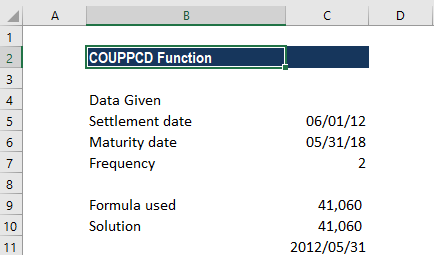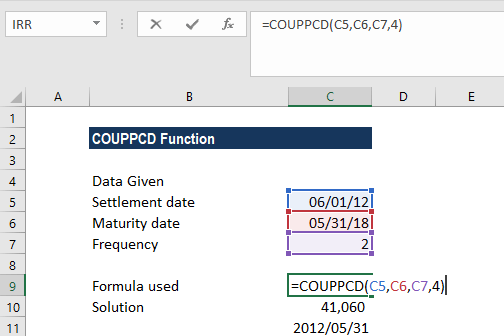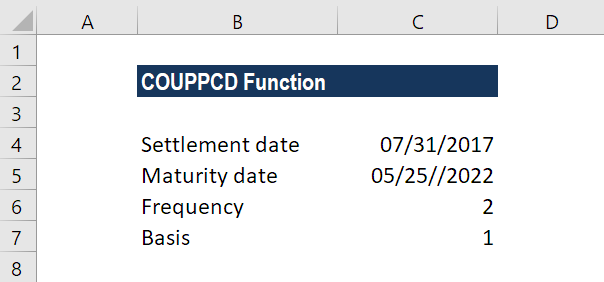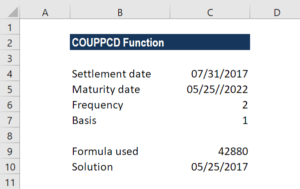COUPPCD Function

Calculates the calendar date of the most recent coupon payment

What is the COUPPCD Function?

The COUPPCD function is a Financial function. It helps calculate the calendar date of the most recent coupon payment. The function returns the result in form of a date.

Formula

=COUPPNCD(settlement, maturity, frequency, [basis])

The COUPPNCD function uses the following arguments:

1. Settlement (required argument) – This is the settlement date of a given security. It is the date after the security is traded to the buyer.
2. Maturity – This is a required argument. It is the date when the security expires.
3. Frequency (required argument) – This is the number of coupon payments per year. The argument can take a value of 1 (annual payment), 2 (semi-annual payments), or 4 (quarterly payments).
4. Basis – (optional argument) – This specifies the day count basis to be used. It uses one of the following values:

BasisDay Count basis
0 or omittedUS(NASD) 30/360
1Actual/actual
2Actual/360
3Actual/365
4European 30/360

The function will default to 0 when omitted. It indicates that the days in the month are counted using the US 30-day method with a 360-day year. When we enter 1 as the basis, the function uses the actual number of days in the month and year. Whereas, when we enter 2, it will count the actual days in the month with a 360-day year, while 3 will assume a 365-day year. When we enter 4 as the basis, it is same as 1 except that it uses the European 30-day method.

How to use the COUPPCD Function in Excel?

To understand the uses of the COUPPCD function, let’s consider a few examples:

Example 1

Let’s see how the COUPPCD function works when we are given the following data:Additionally, we are told that count basis would be 4 as it is European bond. Excel would take the basis as 30/360.

Using the formula =COUPPCD(C5,C6,C7,4), we get the following result:As the result is a number series, we need to format it into a date. The last coupon payment was made on May 31, 2012.

Example 2

As the COUPPCD function doesn’t accept dates in a text format, we need to convert them into a DATE format. Let’s see an example to understand it. Suppose we are given the following data:As we need to find the number of coupon payments that we will receive, using the function, we must first convert the dates given in text format.

The formula to use is =COUPPCD(DATE(2017,7,31),DATE(2022,5,25),2,1).

The COUPPCD function first converted the dates in text format into proper dates and then calculated the number of days. The result is a number series, so we formatted it into a date format.Few notes about the COUPPCD Function:

1. #NUM! error – Occurs in the following scenarios:
• When the settlement date provided is greater than or equal to (≥) the maturity date.
• When the given frequency argument provided by the user is not equal to 1, 2 or 4.
• When the given basis argument is a number other than 0, 1, 2, 3 or 4.
2. #VALUE! – Occurs in the following scenarios:
• When the given settlement date or maturity date is not a valid date. Remember that we need to enter dates in date format or else use the DATE function to convert them into proper dates. The function doesn’t work when dates given are in text format. For example, =COUPPCD(“1/25/2023″,”11/15/2024”,2,1) = #VALUE.
• Any of the arguments given is non-numeric.
3. The COUPPCD function truncates all arguments to integers.

Thanks for reading CFI’s guide to the COUPPCD Excel function. By taking the time to learn and master these functions, you’ll significantly speed up your financial analysis. To learn more, check out these additional CFI resources:

• Excel Functions for Finance
• Advanced Excel Formulas Course
• Advanced Excel Formulas You Must Know
• Excel Shortcuts for PC and Mac

Free Excel Tutorial

To master the art of Excel, check out CFI’s FREE Excel Crash Course, which teaches you how to become an Excel power user.  Learn the most important formulas, functions, and shortcuts to become confident in your financial analysis.

Launch CFI’s Free Excel Course now to take your career to the next level and move up the ladder!